Ex 7.3

Chapter 7 Class 11 Permutations and Combinations
Serial order wise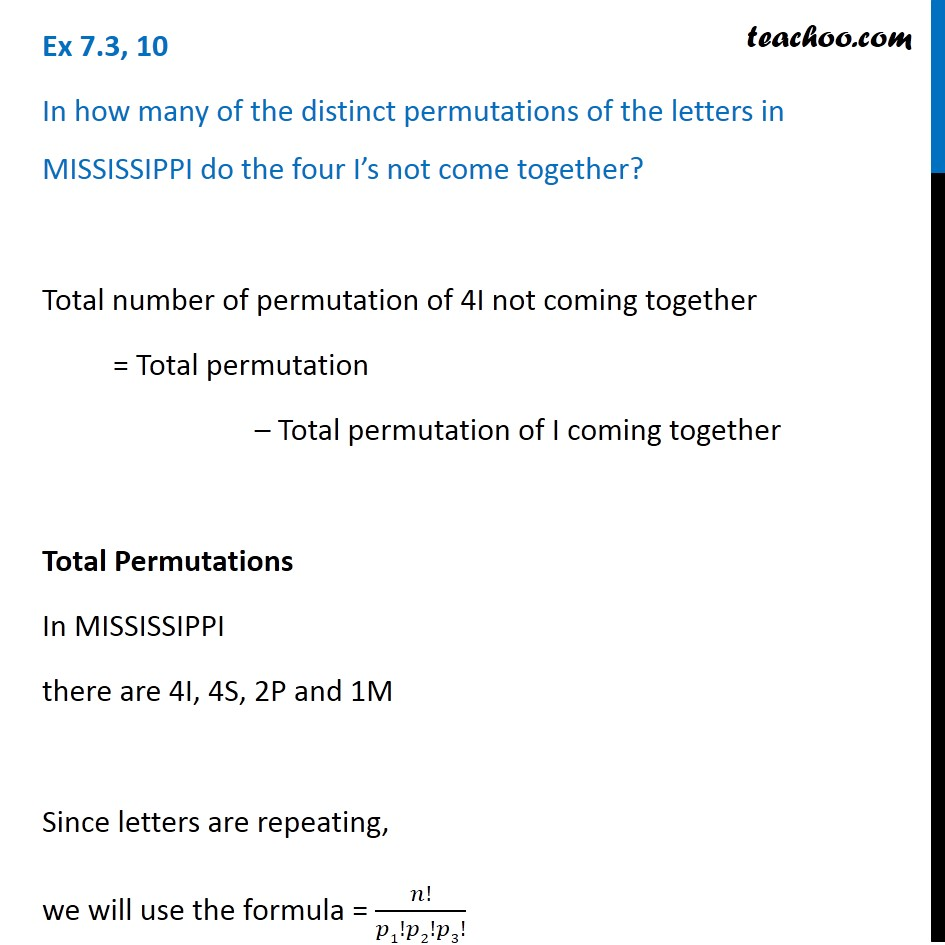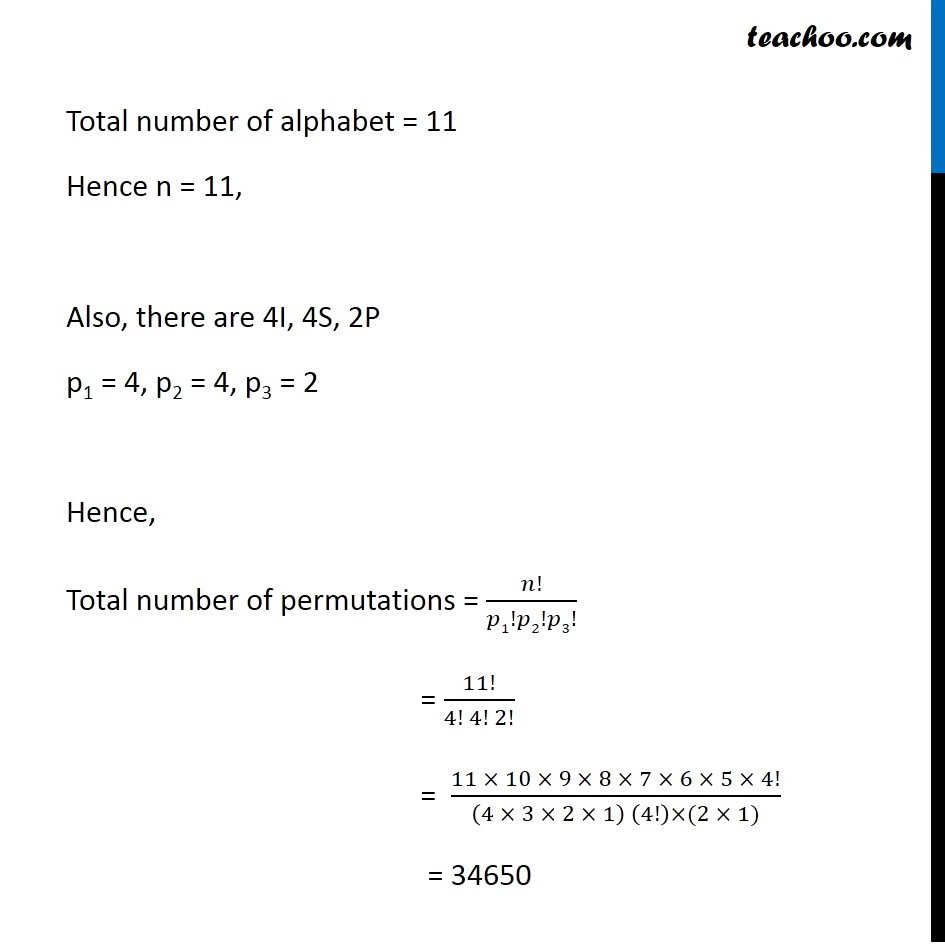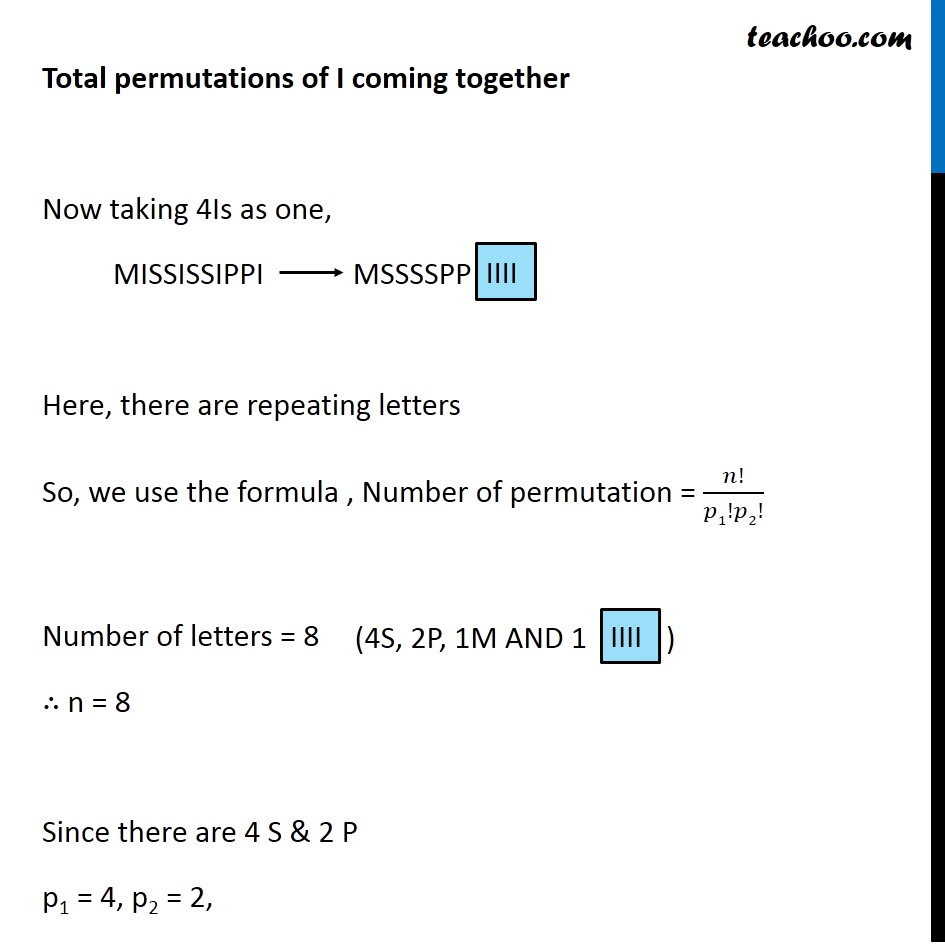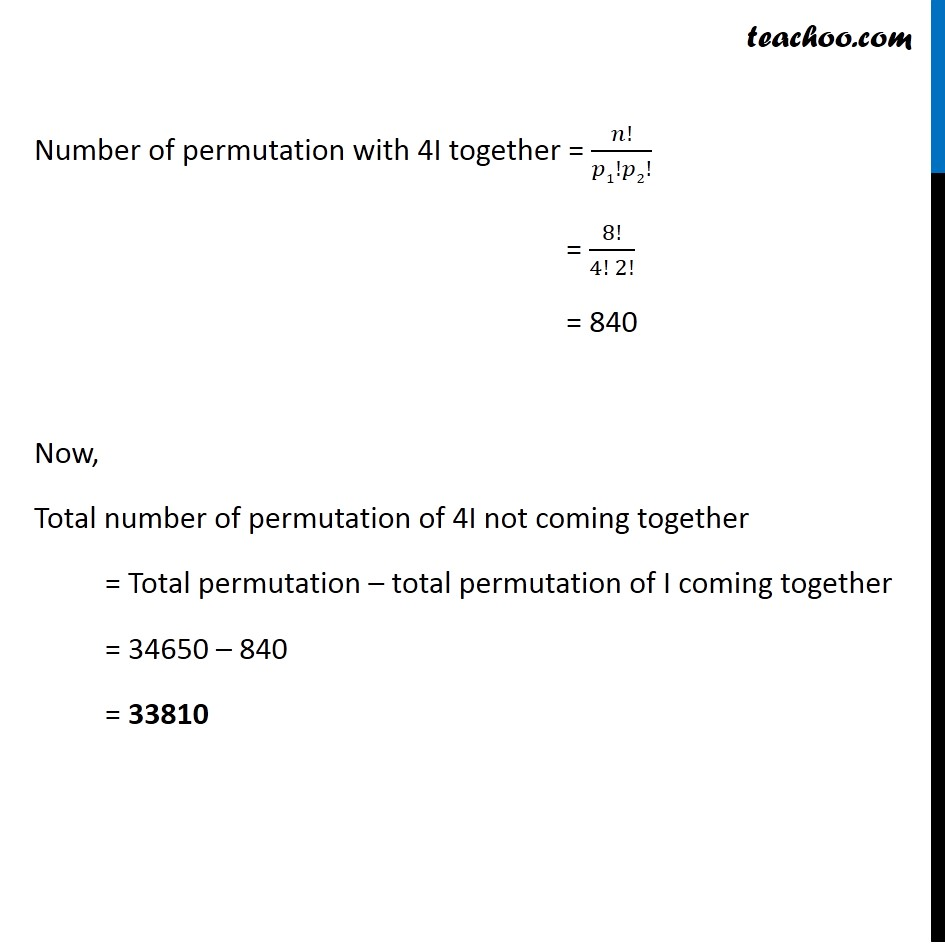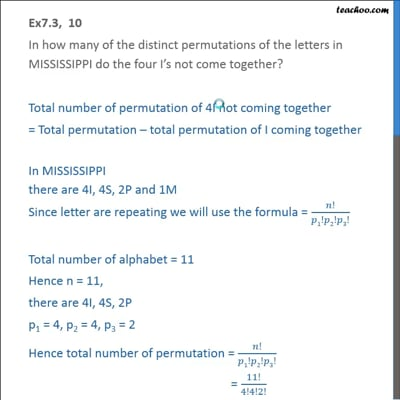This video is only available for Teachoo black users

Introducing your new favourite teacher - Teachoo Black, at only ₹83 per month

### Transcript

Ex 7.3, 10 In how many of the distinct permutations of the letters in MISSISSIPPI do the four I’s not come together? Total number of permutation of 4I not coming together = Total permutation – Total permutation of I coming together Total Permutations In MISSISSIPPI there are 4I, 4S, 2P and 1M Since letters are repeating, we will use the formula = 𝑛!/𝑝1!𝑝2!𝑝3! Total number of alphabet = 11 Hence n = 11, Also, there are 4I, 4S, 2P p1 = 4, p2 = 4, p3 = 2 Hence, Total number of permutations = 𝑛!/𝑝1!𝑝2!𝑝3! = 11!/(4! 4! 2!) = (11 × 10 × 9 × 8 × 7 × 6 × 5 × 4!)/((4 × 3 × 2 × 1) (4!)×(2 × 1)) = 34650 Total permutations of I coming together Now taking 4Is as one, MISSISSIPPI Here, there are repeating letters So, we use the formula , Number of permutation = 𝑛!/𝑝1!𝑝2! Number of letters = 8 ∴ n = 8 Since there are 4 S & 2 P p1 = 4, p2 = 2, Number of permutation with 4I together = 𝑛!/𝑝1!𝑝2! = 8!/(4! 2!) = 840 Now, Total number of permutation of 4I not coming together = Total permutation – total permutation of I coming together = 34650 – 840 = 33810